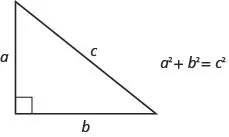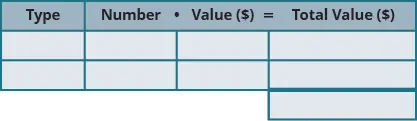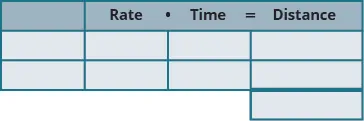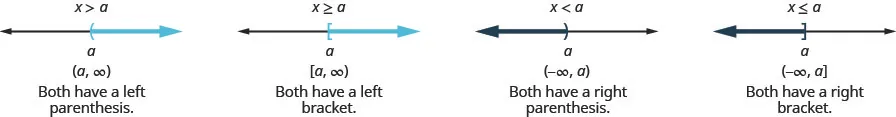Intermediate Algebra 2e

# Key Concepts

### Key Concepts

#### 2.1Use a General Strategy to Solve Linear Equations

• How to determine whether a number is a solution to an equation
1. Step 1. Substitute the number in for the variable in the equation.
2. Step 2. Simplify the expressions on both sides of the equation.
3. Step 3. Determine whether the resulting equation is true.
If it is true, the number is a solution.
If it is not true, the number is not a solution.
• How to Solve Linear Equations Using a General Strategy
1. Step 1. Simplify each side of the equation as much as possible.
Use the Distributive Property to remove any parentheses.
Combine like terms.
2. Step 2. Collect all the variable terms on one side of the equation.
Use the Addition or Subtraction Property of Equality.
3. Step 3. Collect all the constant terms on the other side of the equation.
Use the Addition or Subtraction Property of Equality.
4. Step 4. Make the coefficient of the variable term equal to 1.
Use the Multiplication or Division Property of Equality.
State the solution to the equation.
5. Step 5. Check the solution.
Substitute the solution into the original equation to make sure the result is a true statement.
• How to Solve Equations with Fraction or Decimal Coefficients
1. Step 1. Find the least common denominator (LCD) of all the fractions and decimals (in fraction form) in the equation.
2. Step 2. Multiply both sides of the equation by that LCD. This clears the fractions and decimals.
3. Step 3. Solve using the General Strategy for Solving Linear Equations.

#### 2.2Use a Problem Solving Strategy

• How To Use a Problem Solving Strategy for Word Problems
1. Step 1. Read the problem. Make sure all the words and ideas are understood.
2. Step 2. Identify what you are looking for.
3. Step 3. Name what you are looking for. Choose a variable to represent that quantity.
4. Step 4. Translate into an equation. It may be helpful to restate the problem in one sentence with all the important information. Then, translate the English sentence into an algebra equation.
5. Step 5.
Solve the equation using proper algebra techniques.

6. Step 6. Check the answer in the problem to make sure it makes sense.
7. Step 7. Answer the question with a complete sentence.
• How To Find Percent Change
1. Step 1. Find the amount of change
$change=new amount−original amountchange=new amount−original amount$
2. Step 2. Find what percent the amount of change is of the original amount.
$change is what percent of the original amount?change is what percent of the original amount?$
• Discount
$amount of discount=discount rate·original pricesale price=original amount−discountamount of discount=discount rate·original pricesale price=original amount−discount$
• Mark-up
$amount of mark-up=mark-up rate·original costlist price=original cost+mark upamount of mark-up=mark-up rate·original costlist price=original cost+mark up$
• Simple Interest
If an amount of money, P, called the principal, is invested or borrowed for a period of t years at an annual interest rate r, the amount of interest, I, earned or paid is:
$I=interestI=PrtwhereP=principalr=ratet=timeI=interestI=PrtwhereP=principalr=ratet=time$

#### 2.3Solve a Formula for a Specific Variable

• How To Solve Geometry Applications
1. Step 1. Read the problem and make sure all the words and ideas are understood.
2. Step 2. Identify what you are looking for.
3. Step 3. Name what you are looking for by choosing a variable to represent it. Draw the figure and label it with the given information.
4. Step 4. Translate into an equation by writing the appropriate formula or model for the situation. Substitute in the given information.
5. Step 5. Solve the equation using good algebra techniques.
6. Step 6. Check the answer in the problem and make sure it makes sense.
7. Step 7. Answer the question with a complete sentence.
• The Pythagorean Theorem
• In any right triangle, where a and b are the lengths of the legs, and c is the length of the hypotenuse, the sum of the squares of the lengths of the two legs equals the square of the length of the hypotenuse.#### 2.4Solve Mixture and Uniform Motion Applications

• Total Value of Coins
For the same type of coin, the total value of a number of coins is found by using the model
$number·value=totalvaluenumber·value=totalvalue$
• number is the number of coins
• value is the value of each coin
• total value is the total value of all the coins

• How to solve coin word problems.
1. Step 1. Read the problem. Make sure all the words and ideas are understood.
Determine the types of coins involved.
Create a table to organize the information.
Label the columns “type,” “number,” “value,” “total value.”
List the types of coins.
Write in the value of each type of coin.
Write in the total value of all the coins.2. Step 2. Identify what you are looking for.
3. Step 3. Name what you are looking for. Choose a variable to represent that quantity.
Use variable expressions to represent the number of each type of coin and write them in the table.
Multiply the number times the value to get the total value of each type of coin.
4. Step 4. Translate into an equation.
It may be helpful to restate the problem in one sentence with all the important information. Then, translate the sentence into an equation.
Write the equation by adding the total values of all the types of coins.
5. Step 5. Solve the equation using good algebra techniques.
6. Step 6.
Check the answer in the problem and make sure it makes sense.

7. Step 7. Answer the question with a complete sentence.
• How To Solve a Uniform Motion Application
1. Step 1. Read the problem. Make sure all the words and ideas are understood.
Draw a diagram to illustrate what it happening.
Create a table to organize the information.
Label the columns rate, time, distance.
List the two scenarios.
Write in the information you know.2. Step 2. Identify what you are looking for.
3. Step 3. Name what you are looking for. Choose a variable to represent that quantity.
Complete the chart.
Use variable expressions to represent that quantity in each row.
Multiply the rate times the time to get the distance.
4. Step 4. Translate into an equation.
Restate the problem in one sentence with all the important information.
Then, translate the sentence into an equation.
5. Step 5. Solve the equation using good algebra techniques.
6. Step 6. Check the answer in the problem and make sure it makes sense.
7. Step 7. Answer the question with a complete sentence.

#### 2.5Solve Linear Inequalities

• Inequalities, Number Lines, and Interval Notation
$x>ax≥axax≥ax• Linear Inequality
• A linear inequality is an inequality in one variable that can be written in one of the following forms where a, b, and c are real numbers and $a≠0:a≠0:$
$ax+bc,ax+b≥c.ax+bc,ax+b≥c.$
• Addition and Subtraction Property of Inequality
• For any numbers a, b, and c, if $a
$a+cb+ca−c>b−ca+cb+ca−c>b−c$
• We can add or subtract the same quantity from both sides of an inequality and still keep the inequality.
• Multiplication and Division Property of Inequality
• For any numbers a, b, and c,
$multiply or divide by apositiveifa0,thenacbandc>0,thenac>bcandac>bc.multiply or divide by anegativeifabcandac>bc.ifa>bandc<0,thenac0,thenacbandc>0,thenac>bcandac>bc.multiply or divide by anegativeifabcandac>bc.ifa>bandc<0,thenac
• Phrases that indicate inequalities
$>>$ $≥≥$ $<<$ $≤≤$
is greater than

is more than

is larger than

exceeds
is greater than or equal to

is at least

is no less than

is the minimum
is less than

is smaller than

has fewer than

is lower than
is less than or equal to

is at most

is no more than

is the maximum

#### 2.6Solve Compound Inequalities

• How to solve a compound inequality with “and”
1. Step 1. Solve each inequality.
2. Step 2. Graph each solution. Then graph the numbers that make both inequalities true. This graph shows the solution to the compound inequality.
3. Step 3. Write the solution in interval notation.
• Double Inequality
• A double inequality is a compound inequality such as $a. It is equivalent to $a and $x
$Other forms:ax>bis equivalent toa>xandx>ba≥x≥bis equivalent toa≥xandx≥bOther forms:ax>bis equivalent toa>xandx>ba≥x≥bis equivalent toa≥xandx≥b$
• How to solve a compound inequality with “or”
1. Step 1. Solve each inequality.
2. Step 2. Graph each solution. Then graph the numbers that make either inequality true.
3. Step 3. Write the solution in interval notation.

#### 2.7Solve Absolute Value Inequalities

• Absolute Value
The absolute value of a number is its distance from 0 on the number line.
The absolute value of a number n is written as $|n||n|$ and $|n|≥0|n|≥0$ for all numbers.
Absolute values are always greater than or equal to zero.
• Absolute Value Equations
For any algebraic expression, u, and any positive real number, a,
$if|u|=athenu=−aoru=aif|u|=athenu=−aoru=a$
Remember that an absolute value cannot be a negative number.
• How to Solve Absolute Value Equations
1. Step 1. Isolate the absolute value expression.
2. Step 2. Write the equivalent equations.
3. Step 3. Solve each equation.
4. Step 4. Check each solution.
• Equations with Two Absolute Values
For any algebraic expressions, u and v,
$if|u|=|v|thenu=−voru=vif|u|=|v|thenu=−voru=v$
• Absolute Value Inequalities with $<<$ or $≤≤$
For any algebraic expression, u, and any positive real number, a,
$if|u|
• How To Solve Absolute Value Inequalities with $<<$ or $≤≤$
1. Step 1. Isolate the absolute value expression.
2. Step 2. Write the equivalent compound inequality.
$|u|
3. Step 3. Solve the compound inequality.
4. Step 4. Graph the solution
5. Step 5. Write the solution using interval notation
• Absolute Value Inequalities with $>>$ or $≥≥$
For any algebraic expression, u, and any positive real number, a,
$if|u|>a,thenu<−aoru>aif|u|≥a,thenu≤−aoru≥aif|u|>a,thenu<−aoru>aif|u|≥a,thenu≤−aoru≥a$
• How To Solve Absolute Value Inequalities with $>>$ or $≥≥$
1. Step 1. Isolate the absolute value expression.
2. Step 2. Write the equivalent compound inequality.
$|u|>ais equivalent tou<−aoru>a|u|≥ais equivalent tou≤−aoru≥a|u|>ais equivalent tou<−aoru>a|u|≥ais equivalent tou≤−aoru≥a$
3. Step 3. Solve the compound inequality.
4. Step 4. Graph the solution
5. Step 5. Write the solution using interval notation
Order a print copy

As an Amazon Associate we earn from qualifying purchases.